Electrochemical (2019 VCE) 1) The zinc–cerium battery is a commercial rechargeable battery that comprises a series of cells. During recharging, the cells use energy from wind farms or solar cell panels. During discharging, energy is supplied to electric grids to power local factories and homes. The electrolytes are stored in separate storage tanks, and are pumped into and out of each cell when in use. A membrane separates the two electrodes that are immersed in 1 M methanesulfonic acid, CH3SO3H. A diagram representing a zinc–cerium cell is shown below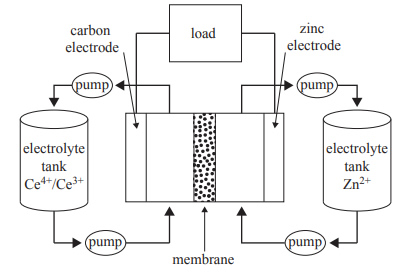The following half-cell reactions occur in the zinc–cerium cell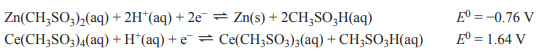a. Write the equation for the overall discharge reaction. b. Identify the oxidising agent during discharging and justify your answer using oxidation numbers Solution c. Determine the theoretical voltage produced by a single cell as it discharges. d. Write the ionic equation for the reaction occurring at the positive electrode during recharging. Solutione. Other than transporting ions between the electrodes, describe one function of the membrane in the zinc–cerium cell. f. Specify one factor that would limit the life of the zinc–cerium cell. Solution g. Experts have regarded the zinc–cerium cell as a hybrid of a fuel cell and a secondary cell. Why would this be the case? Solution2) Which one of the following galvanic cells will produce the largest cell voltage under standard laboratory conditions (SLC)?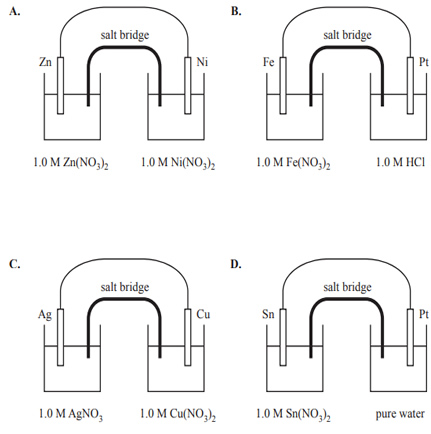Solution3) Consider the following statements about galvanic cells and fuel cells.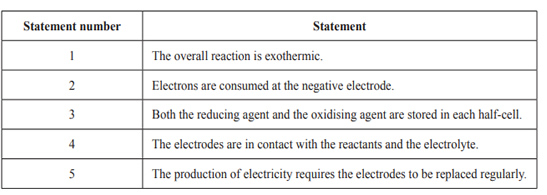Which one of the following sets of statements is correct for both galvanic cells and fuel cells? A. statement numbers 2 and 3 B. statement numbers 1 and 4 C. statement numbers 2, 4 and 5 D. statement numbers 1, 3 and 5   Solution4) At the start of the day, a student set up a galvanic cell using two electrodes: nickel, Ni, and metal X. This set-up is shown in the diagram below.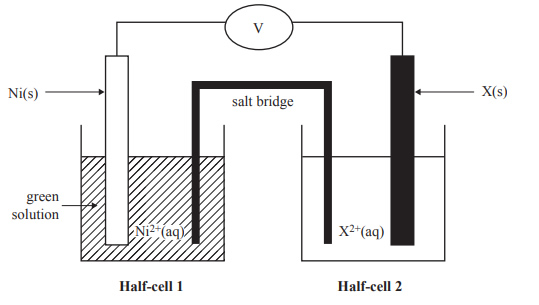Consider the following alternative metals that could be used to replace metal X: 1. zinc, Zn 2. lead, Pb 3. cadmium, Cd 4. copper, Cu At the end of the day, the student checked the colour of the solution in Half-cell 1 and observed that the solution was a darker green colour. Which of the alternative metals could cause the colour of Half-cell 1 to become a darker green? A. metals 1 and 3 B. metals 2 and 4 C. metals 1, 2 and 3 D. metals 3 and 4 Solution